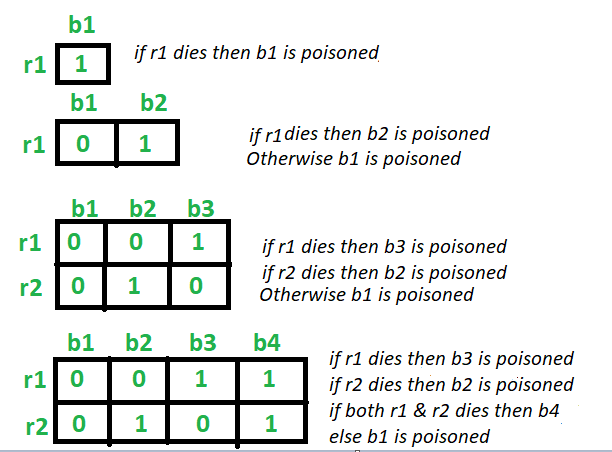Related Articles
Rat and Poisoned bottle Problem
• Difficulty Level : Medium
• Last Updated : 30 Apr, 2020

Given N number of bottles in which one bottle is poisoned. So the task is to find out minimum number of rats required to identify the poisoned bottle. A rat can drink any number of bottles at a time.

Examples:

```Input: N = 4
Output: 2

Input: N = 100
Output: 7
```

## Recommended: Please try your approach on {IDE} first, before moving on to the solution.

Approach:
Let’s start from the base case.

• For 2 bottles: Taking one rat (R1). If the rat R1 drinks the bottle 1 and dies, then bottle 1 is poisonous. Else the bottle 2 is poisonous. Hence 1 rat is enough to identify
• For 3 bottles: Taking two rats (R1) and (R2). If the rat R1 drinks the bottle 1 and bottle 3 and dies, then bottle 1 or bottle 3 is poisonous. So the rat R2 drinks the bottle 1 then. If it dies, then the bottle 1 is poisonous, Else the bottle 3 is poisonous.
Now if the rat R1 does not die after drinking from bottle 1 and bottle 3, then bottle 2 is poisonous.
Hence 2 rats are enough to identify.
• For 4 bottles: Taking two rats (R1) and (R2). If the rat R1 drinks the bottle 1 and bottle 3 and dies, then bottle 1 or bottle 3 is poisonous. So the rat R2 drinks the bottle 1 then. If it dies, then the bottle 1 is poisonous, Else the bottle 3 is poisonous.
Now if the rat R1 does not die after drinking from bottle 1 and bottle 3, then bottle 2 or bottle 4 is poisonous. So the rat R1 drinks the bottle 2 then. If it dies, then the bottle 2 is poisonous, Else the bottle 4 is poisonous.
Hence 2 rats are enough to identify.
• For N bottles:

Minimum number of rats required are = ceil(log2 N))Below is the implementation of the above approach:

## C++

 `// C++ program to implement``// the above approach`` ` `#include ``using` `namespace` `std;`` ` `// Function to find the minimum number of rats``int` `minRats(``int` `n)``{``    ``return` `ceil``(log2(n));``}`` ` `// Driver Code``int` `main()``{``    ``// Number of bottles``    ``int` `n = 1025;`` ` `    ``cout << ``"Minimum "` `<< minRats(n)``         ``<< ``" rat(s) are required"``         ``<< endl;`` ` `    ``return` `0;``}`

## Java

 `// Java program to implement ``// the above approach``class` `GFG``{``    ``public` `static` `double` `log2(``int` `x)``    ``{``        ``return` `(Math.log(x) / Math.log(``2``));``    ``}`` ` `    ``// Function to find the minimum number of rats ``    ``static` `int` `minRats(``int` `n) ``    ``{ ``        ``return` `(``int``)(Math.floor(log2(n)) + ``1``); ``    ``} ``     ` `    ``// Driver Code ``    ``public` `static` `void` `main (String[] args) ``    ``{ ``        ``// Number of bottles ``        ``int` `n = ``1025``; ``     ` `        ``System.out.println(``"Minimum "` `+ minRats(n) + ``                           ``" rat(s) are required"``); ``    ``}    ``} `` ` `// This code is contributed by AnkitRai01`

## Python3

 `# Python3 program to implement ``# the above approach ``import` `math`` ` `# Function to find the ``# minimum number of rats ``def` `minRats(n):``     ` `    ``return` `math.ceil(math.log2(n)); `` ` `# Driver Code `` ` `# Number of bottles ``n ``=` `1025``; ``print``(``"Minimum "``, end ``=` `"")``print``(minRats(n), end ``=` `" "``)``print``(``"rat(s) are required"``)`` ` `# This code is contributed ``# by divyamohan123`

## C#

 `// C# program to implement ``// the above approach``using` `System;`` ` `class` `GFG``{``    ``public` `static` `double` `log2(``int` `x)``    ``{``        ``return` `(Math.Log(x) / Math.Log(2));``    ``}`` ` `    ``// Function to find the minimum number of rats ``    ``static` `int` `minRats(``int` `n) ``    ``{ ``        ``return` `(``int``)(Math.Floor(log2(n)) + 1); ``    ``} ``     ` `    ``// Driver Code ``    ``public` `static` `void` `Main (String[] args) ``    ``{ ``        ``// Number of bottles ``        ``int` `n = 1025; ``     ` `        ``Console.WriteLine(``"Minimum "` `+ minRats(n) + ``                          ``" rat(s) are required"``); ``    ``} ``}`` ` `// This code is contributed by 29AjayKumar`
Output:
```Minimum 11 rat(s) are required
```

My Personal Notes arrow_drop_up